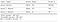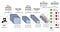# Hand-gesture recognition neural network analysis

Hello there!

In this article, we are going to discuss the influence of some important techniques that are useful when training a neural network, such as hyperparameter tuning, batch normalization, and regularization. Besides, we will also perform a comparison between artificial and convolutional neural networks considering the development of a system that must recognize some basic hand gestures.

To perform this analysis, we will consider the simple SIGNS dataset, which has 1300 pictures of hand signs representing numbers from 0 to 5. An example of each possible class is shown in the figure below. This dataset is taken from the Coursera course Convolutional Neural Networks, by Professor Andrew Ng.

The idea is to develop a neural network that receives as input one hand-gesture image and outputs which number it represents. We divided the training and test set as follows:

• Training set: 1080 pictures (64 x 64 pixels) of signs representing numbers from 0 to 5 (180 pictures per number).
• Test set: 120 pictures (64 x 64 pixels) of signs representing numbers from 0 to 5 (20 pictures per number).

The code that was used to generate the results can be found here, at my Github.

# Baseline Neural Network Model

Let’s train our first model, which will give us an initial value of accuracy. The architecture of this neural network is shown in the figure below, where we have two hidden layers, with 25 and 12 neurons for the first and second hidden layers, respectively, and 307.615 parameters to be trained.Architecture of the baseline neural network.

We also used the following hyperparameters: learning rate = 0.0001, batch size = 32, epochs =1000, and the Gradient descent (with momentum) optimizer. The loss and the accuracy for the training and test sets according to the number of epochs are shown in the figure below:

As it can be seen, the loss decreases and the accuracy of both train and test set increases as the number of epochs increases. Besides, it is also possible to see that model is overfitting since the training accuracy is much higher than the test accuracy. At the end of the training, we acquired an accuracy of 0.882 for the training set and 0.792 for the test set, which corresponds to a difference of approximately 10%, suggesting that the network is indeed overfitting. It took 160 seconds to train the model.

Throughout the rest of this article, we will try to improve this obtained value of accuracy by testing different techniques.

# Hyperparameters

Now, let’s try to get a better accuracy result by initially using hyperparameter tuning. There are many hyperparameters that can be tuned when training a neural network, such as learning rate, batch size, number of hidden units, epoch, number of layers, and so on.

The learning rate is a hyperparameter that controls how much to change the model in response to the estimated error each time the model weights are updated. Choosing the learning rate is challenging as a value too small may result in a long training process that could get stuck, whereas a value too large may result in learning a sub-optimal set of weights too fast or an unstable training process.

The batch size defines the number of samples that will be propagated through the network. For instance, we have 1080 training samples and we want to set up a batch size equal to 32. The algorithm takes the first 32 samples (from 1st to 32nd) from the training dataset and trains the network. Next, it takes the second 32 samples (from 33th to 64h) and trains the network again. We can keep doing this procedure until we have propagated all samples through the network. Besides, in this case, there will be 34 (1080/32) batches.

Another interesting hyperparameter that can be tuned is the number of epochs, which is defined as the number of times that the learning algorithm will work through the entire training dataset. One epoch means that each sample in the training dataset has had an opportunity to update the internal model parameters. An epoch is comprised of one or more batches. For the example given above, 1 epoch will have 34 batches, since there are 1080 training samples and the batch size is 32.

In this article, we will focus on tuning the learning rate, since it is the most important hyperparameter, and the number of hidden units of the first layer. We used the Random Search and tested 6 different model configurations by varying the number of inputs of the first hidden layer from 20 to 40 (using a 5 step), and the learning rate from 0.0001 to 0.001.

The model with the best training accuracy was the one that used 35 hidden units in the first layer and a learning rate of 0.001132. The results of this model when training with 500 epochs and a batch size of 32 can be seen in the figure below.

This model achieved a training accuracy of 0.988 and testing accuracy of 0.867, which has a better performance than the baseline model previously presented. However, it is clearly overfitting, since the difference between training and test results are approximately 13 %.

# Batch Normalization

Training deep neural networks with tens of layers is challenging as they can be sensitive to the initial random weights and configuration of the learning algorithm. One possible reason for this difficulty is the distribution of the inputs to layers deep in the network may change after each minibatch when the weights are updated. This can cause the learning algorithm to forever chase a moving target.

Batch normalization is a technique for training very deep neural networks that standardizes the inputs to a layer for each minibatch. This technique accelerates training, in some cases by halving the number of epochs (or better), and provides some regularization effect, reducing generalization error.

In this work, we will apply batch normalization before the activation function of the previous layer, as suggested in the original paper that introduced this technique. Furthermore, we will use the same architecture presented in the Baseline Neural Network Model section but with 35 units in the first hidden layer, and a learning rate of 0.001132 (both changes provided better results as pointed out in the Hyperparameter section). Besides, we also will use 500 epochs, and a batch size of 32 in the training process. The figure below shows the results for the loss and accuracy for both train and test sets:

For this model, we acquired an accuracy of 1.00 for the training set and 0.883 for the test set, which corresponds to a difference of approximately 12 %. This model took 92,57 seconds to be trained.

This model achieved the best accuracy so far, exceeding the result related to the test set from the model trained in the Hyperparameter section by 2%, and was the fastest to be trained, since we only used 500 epochs to train. However, we can still observe that the model is overfitting.

# Regularization

Regularization is a technique that makes slight modifications to the learning process such that the model generalizes better. This in turn improves the model’s performance on the unseen data as well, reducing problems related to overfitting.

The standard way to use regularization is through L2 regularization (defined by a λ value), which works by adding a term to the error function used by the training algorithm. This additional term penalizes large weight values.

We also used hyperparameter tuning in the λ value applied in the first and second layers, and the model with the best performance was the one that used λ1 = 0.01977 and λ2 =0.00894, where λ1 and λ2 are related with the first and second hidden layers, respectively.

The figure below shows the plot of the loss and accuracy of the training and test accuracy related to the number of epochs trained. We used learning rate =0.001132, batch size = 32, and epoch = 500.

In this hyperparameter tuning, we set to maximize the accuracy of the test set, and we achieved an accuracy of 0.9852 for the training set and 0.874 for the test set. Both results are better than the two first developed models, but it is worse than the Batch Normalization model.

However, it is interesting to note that it may have another configuration of L2 values that results in better values of accuracy.

# Convolutional Neural Network

A Convolutional Neural Network (ConvNet/CNN) is a Deep Learning algorithm that can take in an input image, assign importance (learnable weights and biases) to various aspects/objects in the image, and be able to differentiate one from the other.A CNN sequence to classify handwritten digits.

This type of neural network is better than the traditional neural network when it is necessary to detect any object in an image, which is the case of the application proposed in this paper. CNNs gives much better accuracy because they are able to automatically extract visual features from the image. To see more details of the Convolutional Neural Network, feel free to read the article from Sumit Saha, which gives more details about how they work.

In this article, we will train a CNN model to get better accuracy for our application. The architecture of the convolutional neural network is shown in the Figure below.

The types of layers are different from what we saw until now, but it is interesting to note that there are only 65.702 trainable parameters, which is much less than we have in the model previously trained.

In the training process, we used the following hyperparameters: learning rate = 0.001, batch size = 32, epochs =75, and the Adam optimizer. The loss and the accuracy for the training and test sets according to the number of epochs are shown in the figure below:

This model achieved a training accuracy of 0.993 and an impressive testing accuracy of 0.943, which has the best performance of all previous models! Also, the difference between training and test results is only 5%, which is also the lowest value that we achieved. Thus, this system does not overfit much. The downside is that the time spent to train this model was 251 seconds, which is much higher than the models previously trained.

In this way, we can conclude that this CNN model provided the best results for this application. Besides, we could even improve the accuracy by performing hyperparameters tuning, regularization, and other techniques in the CNN architecture.

# Conclusion

In this article, we provided an extensive analysis of techniques that can be employed to improve the accuracy results of neural networks, such as hyperparameter tuning, batch normalization, and regularization, where it was possible to observe that the model achieved better results when any of these techniques were applied.

We also introduce convolutional neural networks, which are very useful when working with images. The application proposed in this paper achieved the best performance when using a CNN model, proving that this type of neural network is very useful for these types of applications.

I hope that you enjoyed reading and see you next time!